Function Repository Resource:

# SomosSequence

Get elements of the Somos sequences

Contributed by: Sander Huisman
 ResourceFunction["SomosSequence"][n,k] give the nth term for the k-order Somos sequence.

## Details

The Somos sequence is defined recursively. For fixed order k, the nth term is given as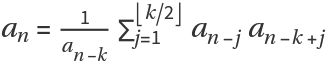, where aj=1 for 0<=j<=k-1.

## Examples

### Basic Examples (2)

Get the 14th term for the 4th order Somos sequence:

 In:=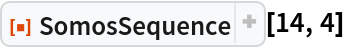Out=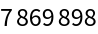Calculate the first 14 elements of the 4th-order Somos sequence:

 In:=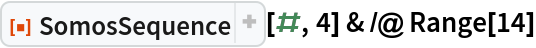Out=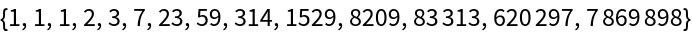### Scope (1)

Elements need not be integers:

 In:=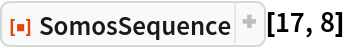Out=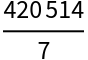### Neat Examples (2)

Plot the 4th-order Somos sequence versus the 5th-order Somos sequence:

 In:=Out=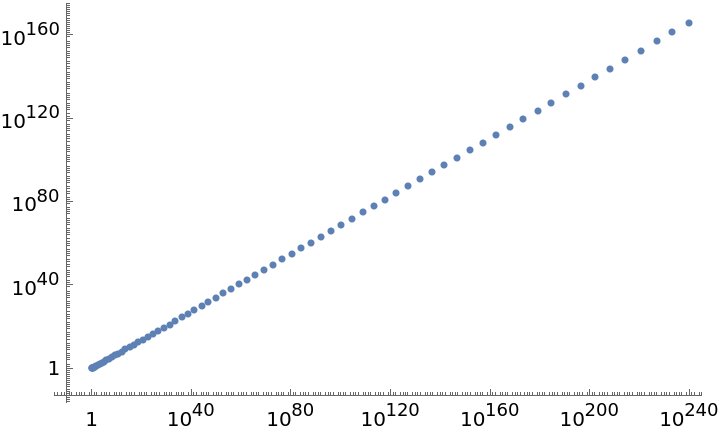Find the scaling exponent:

 In:=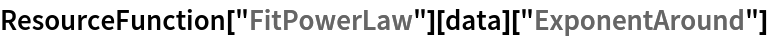Out=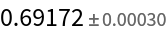SHuisman

## Version History

• 1.1.0 – 08 May 2023
• 1.0.0 – 24 April 2023